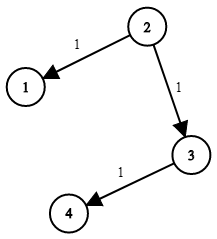# Network Delay Time - (Leetcode 743) - Coding Interview QuestionMani
Educating everyone with the beauty of programming!!

You are given a network of `n` nodes, labeled from `1` to `n`. You are also given `times`, a list of travel times as directed edges ```times[i] = (ui, vi, wi)```, where `ui` is the source node, `vi` is the target node, and `wi` is the time it takes for a signal to travel from source to target.

We will send a signal from a given node `k`. Return the time it takes for all the `n` nodes to receive the signal. If it is impossible for all the `n` nodes to receive the signal, return `-1`.

Example 1:```Input: times = [[2,1,1],[2,3,1],[3,4,1]], n = 4, k = 2
Output: 2
```

Example 2:

```Input: times = [[1,2,1]], n = 2, k = 1
Output: 1
```

Example 3:

```Input: times = [[1,2,1]], n = 2, k = 2
Output: -1
```

Constraints:

• `1 <= k <= n <= 100`
• `1 <= times.length <= 6000`
• `times[i].length == 3`
• `1 <= ui, vi <= n`
• `ui != vi`
• `0 <= wi <= 100`
• All the pairs `(ui, vi)` are unique. (i.e., no multiple edges.)

Solution:

``````1
2
3
4
5
6
7
8
9
10
11
12
13
14
15
16
17
18
19
20
21
22
23
24
25
26
27
28
29
30
31
32
33
34
35
36
37
38
39
40
class Solution {
public int networkDelayTime(int[][] times, int n, int k) {
Map<Integer, List<int[]>> graph = new HashMap<>();
for (int i = 1; i <= n; i++) {
graph.put(i, new ArrayList<>());
}

for (int[] t : times) {
}

Queue<int[]> q = new PriorityQueue<>((a, b) -> a - b);
Map<Integer, Integer> res = new HashMap<>();
while (q.size() != 0) {
int[] cur = q.poll();
if (res.containsKey(cur)) {
continue;
}
//We try to add the same element to the queue.
//But only the cur having minimum value will be considered because
//of the above containsKey condition.
res.put(cur, cur);
for (int i[] : graph.get(cur)) {
q.add(new int[]{i, cur + i});
}

}

if (res.size() != n) {
return -1;
}

int time = 0;
for (int t : res.values()) {
time = Math.max(time, t);
}
return time;
}
}
``````
Drop your comments below for any questions or comments. For full list of must learn top interview questions Click Here: Must Learn Top Interview Questions

Rating: